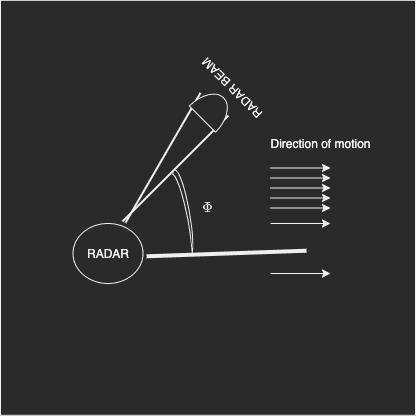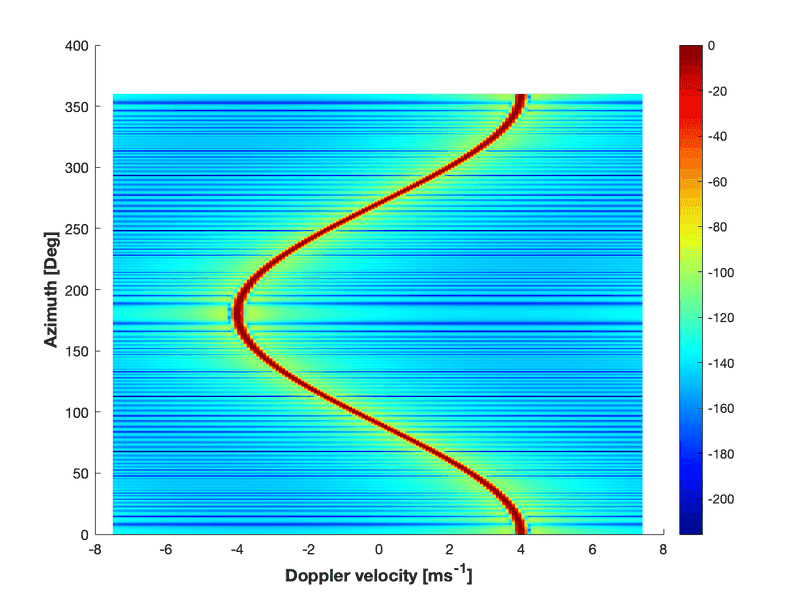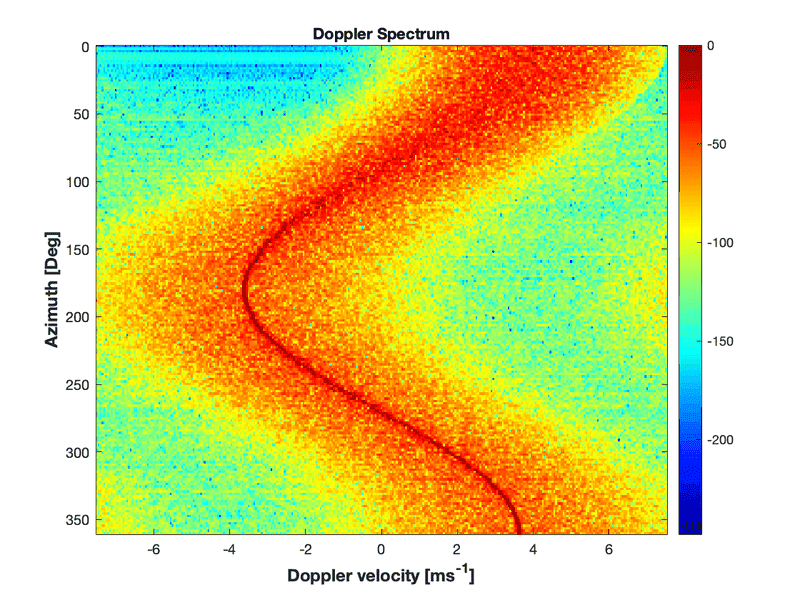# Rotating radar time domain data

I want to simulate the time domain data for a rotating radar. I assume that the space around the radar is filled up with a very big extended object and it moves with a constant speed in one direction. Picture attached.I don't take range information here. I am only concerned about the velocity as in all ranges I consider the velocity to be constant.

My approach:

I consider first when the radar is not rotating and it is directed at ϕ=0 (exactly the same direction of the motion of the target). In that case, the time domain data looks like the following.

$$s(t) = e^{j \frac{4\pi}{\lambda }\mu t}$$

Where $\mu$ is the velocity of the target. The term $\lambda$ is the wavelength of the radar.

If I observe the phase of this signal carefully, it is just an integral over the velocity with respect to time.

$$Phase(s(t)) \propto \int \mu dt$$

This way I did my thought experiment. If I see the target from a rotating radar, the radial velocity I receive from different directions in space would have a cosine dependence.

$$v_r(t) = cos(\phi(t)) \mu$$

Here $v_r$ is the radial velocity that radar receives. The term $\phi(t)$ is a function of $t$ and the rotation speed $\Omega$ . This is basically the angle subtended by the radar beam with the target motion direction at a given time.

$$\phi(t) = \phi_{target} - (\Omega t + \phi_0)$$

Where $\phi_0$ is the initial angle of observation and $\phi_{target}$ is the target motion direction.

Keeping this in mind I performed the following integral

$$\int cos(\phi(t)) \mu dt$$

Which is

$$\frac{-1}{\Omega} sin(\beta_{target} - (\Omega t + \phi_0))$$

So, my time-domain data becomes,

$$s(t) = e^{j \frac{-4\pi}{\lambda \Omega} sin(\beta_{target} - (\Omega t + \phi_0))}$$

I did one simulation with the target motion direction as $\phi_{wind} = 0$ and $\phi_0 = 0$ . I have data for the entire rotation and I plot a micro-doppler spectrogram. I have $167\times360$ points for one rotation. So, I do Doppler FFT processing for every one degree of $\phi$ (167 points DFT) and plot it. Figure attached.Although it looks correct, I need a second eye who verifies my process here and if I am doing it alright physically. Physically radar receives the radial velocity and with that in mind, I formulated the phase of the time domain signal.

Tom.G
That curve looks more like the received signal level from the target than the frequency shift from a constant-speed target.

Baluncore
@tworitdash
1. Is the radar fixed in one place ?
2. Does the target move in a straight line with a fixed velocity ?
3. Is the target a single point reflector, or is it a flock of scatterers that surround the radar in all directions like a local dust storm or snow storm ?

@tworitdash
1. Is the radar fixed in one place ?
2. Does the target move in a straight line with a fixed velocity ?
3. Is the target a single point reflector, or is it a flock of scatterers that surround the radar in all directions like a local dust storm or snow storm ?
1. The radar is fixed physically at one place but its beam, which is narrow (1-degree beamwidth) is rotating from 0 to 360 deg in this case in the azimuth.
2. The target here is an extended target (yes, like a cloud) and it moves with a constant velocity in a constant direction (here it is $\phi = 0$ or you can say x-axis direction in the 2D plane)
3. For this question I consider monochromatic velocity, but at the same time, I consider a big cloud surrounding the radar to make the question simple. However, in my actual model, I use a group of scatterers making a Gaussian spectrum for velocity. You should consider points 1 and 2 for this question. I can relate it with my own model after I see that it is in fact physically viable.

That curve looks more like the received signal level from the target than the frequency shift from a constant-speed target.
This is the spectrum function plotted and not the received signal. I didn't understand fully what you said. Did you mean it looks like the spectrum of a moving target but with an acceleration? Or do you mean a target that is rotating?

Baluncore
Yes, your plot is correct for a cloud moving towards the radar from azimuth zero, with a velocity of 4 m/sec.

The azimuth function will be simple; doppler velocity = cloud velocity * Cos( azimuth );

I note that with fixed azimuth, for a very narrow point beam, all returns from any range will have the same relative velocity, and so will have the same doppler shift.

•tworitdash
Yes, your plot is correct for a cloud moving towards the radar from azimuth zero, with a velocity of 4 m/sec.

The azimuth function will be simple; doppler velocity = cloud velocity * Cos( azimuth );

I note that with fixed azimuth, for a very narrow point beam, all returns from any range will have the same relative velocity, and so will have the same doppler shift.
Thank you for looking at it. I was confused some days ago about how to model the phase in this case. It seems that the math is correct. Now, I can implement a similar transformation to my Gaussian spectrum model (which is not monochromatic).

Baluncore
Now that I think about it, since range is NOT determined, can it really be called RADAR, maybe it is just RAD.

••Klystron, berkeman and tworitdash
Now that I think about it, since range is NOT determined, can it really be called RADAR, maybe it is just RAD.
Haha Yes, it is just RAD I think for now. However, the actual problem is more complex than this. I need to add range information soon and also I will make it 3D where I need to consider the elevation angle as well. I also need to have clouds at different places and not everywhere. So, a lot of things to consider after this. I am simulating for a pulse radar now as you mentioned, but endgame is FMCW of course :P

•Baluncore
Tom.G
This is the spectrum function plotted and not the received signal. I didn't understand fully what you said. Did you mean it looks like the spectrum of a moving target but with an acceleration? Or do you mean a target that is rotating?
Ahh. Lack of data led me to a wrong conclusion. I expected a solid extended target with the RADAR beam rotating. Since the target is a constellation of micro-targets in random motion I will bow out and leave it to those more knowledgable in the field.
Sorry for any confusion.

•Delta2 and tworitdash
sophiecentaur
Gold Member
2020 Award
Yes, your plot is correct for a cloud moving towards the radar from azimuth zero, with a velocity of 4 m/sec.
I don't understand that. The positive and negative values for velocity would imply that the 'cloud' of objects is moving past (around) the observer in a direction from 0 Az to 180Az, some are decreasing in distance and some are increasing in distance. Is that a naive interpretation?
Perhaps I'm not actually disagreeing with you.

Baluncore
Is that a naive interpretation?
Perhaps I'm not actually disagreeing with you.
A wide cloud of reflectors flowing horizontally in one direction, surrounds and flows past a CW radar. At zero elevation, the doppler shift of the received ensemble signal is dependent on the relative azimuth to the stream of the radar pencil beam. It is resolving a component of the stream velocity.

"Towards the radar from azimuth zero" = "Away from the radar to azimuth 180°".

The distance to any individual reflectors is unimportant as they all have the same velocity and so the same doppler shift. While the radar is surrounded by the cloud the r-4 radar equation suggests near returns will dominate the ensemble.

sophiecentaur
Gold Member
2020 Award
"Towards the radar from azimuth zero" = "Away from the radar to azimuth 180°".
How can there not be a change of sign of the doppler velocity as cloud particles go past the observer. I'm sure we must be talking at cross purposes. Your "towards" and "away" imply different signs for the doppler velocity, which the OP's graph shows. I think I picked up on your statement because you didn't imply the fact that the (large ) cloud is going past and not just towards, which accounts for the positive and negative values on the graph.

The OP was concerned about the fact that the graph doesn't include random motions of the particles in the simulation. I would imagine that thermal (brownian?) motion would be small for water droplets, compared with the air molecules, in view of the large ratio of masses. Otoh, turbulence could be relevant under some conditions.

Baluncore
I'm sure we must be talking at cross purposes.
In post #3 we determined it was a surrounding cloud.
Then I needed to describe the direction component of the wind velocity. A North wind gets confusing because it blows towards the South. I used "towards the radar from azimuth zero", which simply eliminated the need for knowing where North was relative to azimuth zero.

sophiecentaur
Gold Member
2020 Award
In post #3 we determined it was a surrounding cloud.
Then I needed to describe the direction component of the wind velocity. A North wind gets confusing because it blows towards the South. I used "towards the radar from azimuth zero", which simply eliminated the need for knowing where North was relative to azimuth zero.
Yes, I'd absolutely go along with that. But, as the French man who was just given one egg for his breakfast, said: "Un oeuf is not always enough". Some of us appreciate getting things twice.I liked the discussion. @Baluncore is right in understanding my problem and therefore, it is an extended cloud moving in one direction. However, now I have a more interesting problem and I think I solved it but I don't know how it worked. I need help in comprehending the mathematics behind it. The reality remains the same. extended cloud and it is moving in one direction that is zero azimuth. However, to make @sophiecentaur happy, I now consider different drop sizes in the cloud that move at different velocities. I assume the spectrum of this velocity of drops takes a Gaussian shape with a mean velocity ($\mu$) and a standard deviation ($\sigma$).

$$S(v) = \frac{1}{\sqrt{2 \pi \sigma^2}} e^{\frac{-(v - \mu)^2}{2 \sigma^2}}$$

If I do an IFFT of the square root of this I get the time domain output.

After IFFT,

$$s(t) = A(t) e^{\Psi(t)}$$

However, this formula is valid if the radar is looking at the same direction of motion as the particles. I need to use a similar kind of transformation in the phase of this time-domain signal as I showed in my original post to have the information of the rotation.

There it was simple. It was monochromatic and I multiplied $cos( \phi(t) )$ to it and integrated it to get the phase of the time domain signal. Here, it is not monochromatic. That is why I can't perform this integration directly.

if you see my last equation in the original post (it is missing a $\mu$ in the phase), you can find that the phase doesn't change linearly with time. That is the reason why I thought when doing an IFFT here for the Gaussian, probably the phase is already changing linearly with time ($\psi(t) \propto t$). So, I used the same transformation from my original post, but I divide it with the time axis.

$$s(t) = A(t) e^{\Psi(t) \frac{sin(\Omega t)}{\Omega t}}$$

If this is my time-domain signal and I perform a spectrogram, I get the following result for $\mu = 4 [m/s]$ and $\sigma = 1 [m/s]$ . This is the desired result but it is not very symmetric. Azimuth zero and 360 should show the same spectrum, but it is different. It is probably due to this transformation in phase.Last edited:
•sophiecentaur
Baluncore
•I know how it should look like. however, I am not able to do the mathematics when I need to use the rotation information in the time domain. That is doing something in the phase of the original Gaussian signal $\Psi(t)$.